# Fibonacci Numbers & The Golden Ratio From The Quadratic Formula

in StemSocial2 years ago

Hi there. In this math post I cover the topic of golden ratio and its relation with the Fibonacci sequence of numbers.

I use LaTeX and Quicklatex.com for rendering the math text.Pixabay Image Source

## The Fibonacci Sequence Of Numbers

The Fibonacci sequence of numbers is a well known sequence of numbers. It starts with 0 and 1 as the first two numbers. The third number is the sum of 0 and 1 which is 1. The fourth number is the sum of the third number which is 1 and the second number which is 1. Obtaining the next number in the Fibonacci sequence involves taking the sum of the previous two numbers.

0, 1, 1, 2, 3, 5, 8, 13, 21, 34, ...

In math notation we would have: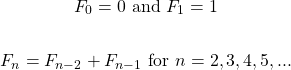This is a recursive relation as obtaining the n-th Fibonacci number depends on the previous Fibonacci numbers.

Example

What is the seventh Fibonacci number in this sequence?

The seventh Fibonacci number is the sum of the sixth Fibonacci number and the fifth Fibonacci number.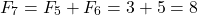Eight is the seventh Fibonacci number.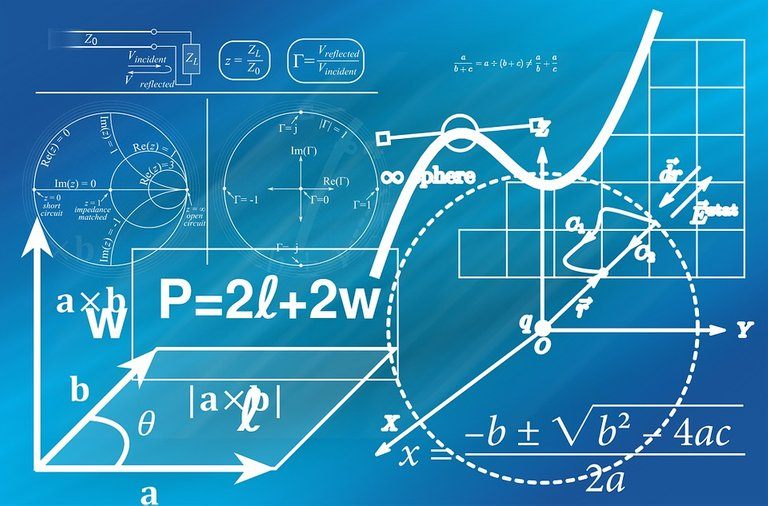Pixabay Image Source

## Obtaining The Golden Ratio

So far we have the recursive relation for obtaining the n-th Fibonacci number.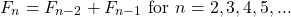With index shifting, the above can be rewritten such that there is no minus sign.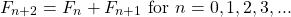Let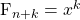. The Fibonacci relation from above is now into: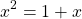Setting the quadratic equal to zero yields: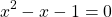The quadratic equation is not factorable. Solving this quadratic requires the quadratic formula. For this case `a = 1`, `b = -1` and `c = -1`.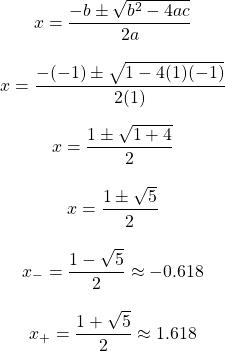The answer of interest is the plus answer of the approximate value of 1.618. This represents the ratio of a Fibonacci number divided by the previous Fibonacci number.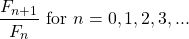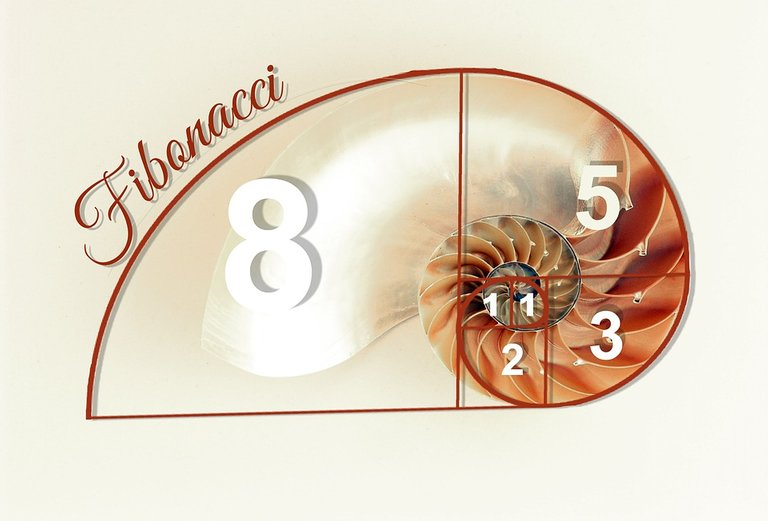Pixabay Image Source

There is a lot more information on the golden ratio not covered in this post. I wanted to cover the derivation of the golden ratio with the use of Fibonacci numbers and the quadratic formula.

Sort:

I never knew the link between the golden ratio and Fibonacci numbers, so thank you for a technical but straightforward post.

Also have you ever heard that the inspiration behind Fibonacci numbers was to model how rabbit populations can grow under ideal situations. Its amazing how a curious mind can come up with a discovery with far greater applications than intended.

I have not heard about the rabbit populations inspiration. Would have to look into that.Thanks for your contribution to the STEMsocial community. Feel free to join us on discord to get to know the rest of us!

Please consider supporting our funding proposal, approving our witness (@stem.witness) or delegating to the @stemsocial account (for some ROI).

Please consider using the STEMsocial app app and including @stemsocial as a beneficiary to get a stronger support.